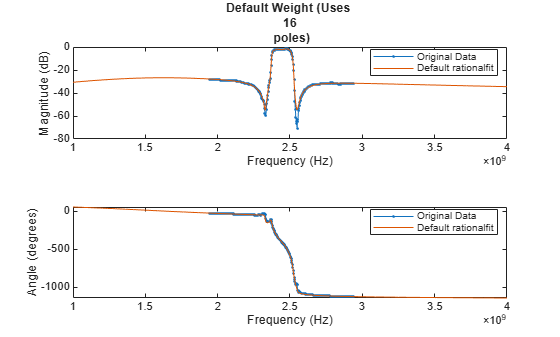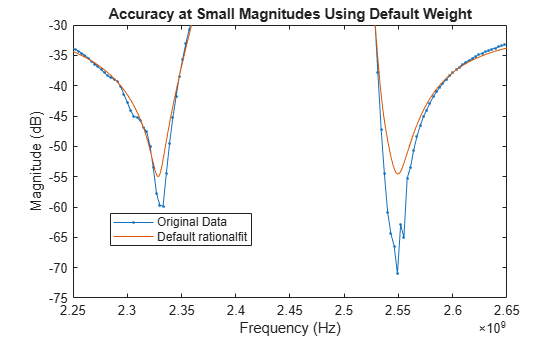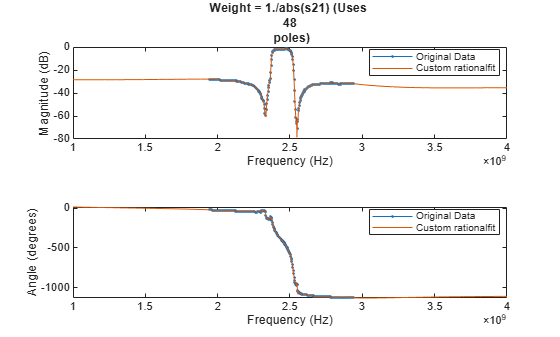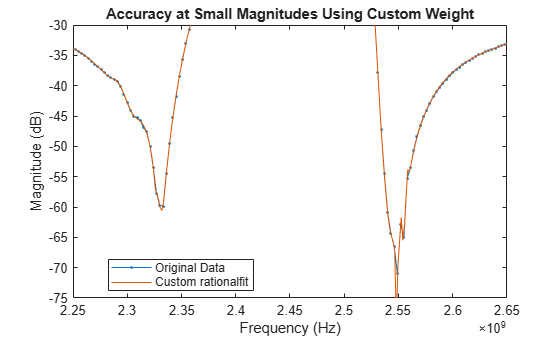## Using the 'Weight' Parameter with rationalfit

This example shows how to use the 'Weight' parameter to improve the quality of the output of `rationalfit`. By default, the `rationalfit` function minimizes the absolute error between the data and the rational function, treating all data points equally. When it is useful to emphasize some of the data points more than the others, use the 'Weight' parameter.

If the magnitude of the input data has a large dynamic range, it is often useful to be more concerned with the relative error at each data point, rather than the absolute error at each data point, so that the data points with relatively smaller magnitudes are fit accurately. The common way to do this is to set the 'Weight' parameter to `1./abs(data)`.

First, read in the saw filter data contained in the file `sawfilter.s2p`, and plot the `S21` data. Next, use the `rationalfit` function to fit a rational function to the `S21` data, with the 'Weight' parameter set to its default value, and visually compare the results to the original data. Lastly, use `rationalfit` again, this time specifying the 'Weight' parameter to be `1./abs(S21)`, and see if the result improves.

### Read and Visualize the Data

```S = sparameters('sawfilter.s2p'); figure subplot(2,1,1) rfplot(S,2,1,'db') subplot(2,1,2) rfplot(S,2,1,'angle') ```### Analyze the Output of rationalfit when Using the Default Value for 'Weight'

Use the `rfparam` function to extract the `S21` values, and then call `rationalfit`.

```s21 = rfparam(S,2,1); datafreq = S.Frequencies; defaultfit = rationalfit(datafreq,s21); ```

Use the `freqresp` function to calculate the response of the output of `rationalfit`.

```respfreq = 1e9:1.5e6:4e9; defaultresp = freqresp(defaultfit,respfreq); ```

Compare the original data against the frequency response of the default rational function calculated by `rationalfit`.

```subplot(2,1,1) plot(datafreq,20*log10(abs(s21)),'.-') hold on plot(respfreq,20*log10(abs(defaultresp))) hold off xlabel('Frequency (Hz)') ylabel('Magnitude (dB)') defaultnpoles = numel(defaultfit.A); defaultstr = ['Default Weight (Uses ',num2str(defaultnpoles),' poles)']; title(defaultstr) legend('Original Data','Default rationalfit','Location','best') subplot(2,1,2) plot(datafreq,unwrap(angle(s21))*180/pi,'.-') hold on plot(respfreq,unwrap(angle(defaultresp))*180/pi) hold off xlabel('Frequency (Hz)') ylabel('Angle (degrees)') legend('Original Data','Default rationalfit','Location','best') ```While the output of `rationalfit` is not awful, it does not match the regions in the data that are very small in magnitude.

```figure plot(datafreq,20*log10(abs(s21)),'.-') hold on plot(respfreq,20*log10(abs(defaultresp))) hold off axis([2.25e9 2.65e9 -75 -30]) xlabel('Frequency (Hz)') ylabel('Magnitude (dB)') title('Accuracy at Small Magnitudes Using Default Weight') legend('Original Data','Default rationalfit','Location','best') ```Using the 'Weight' parameter to make that data relatively more important can help the accuracy of the fit.

### Analyze the Output of rationalfit when Using a Custom Value for 'Weight'

By using a 'Weight' of `1./abs(s21)`, `rationalfit` will minimize the relative error of the system, instead of the absolute error of the system.

```customfit = rationalfit(datafreq,s21,'Weight',1./abs(s21)); customresp = freqresp(customfit,respfreq); ```
```Warning: Achieved only -39.7 dB accuracy with 48 poles, not -40.0 dB. Consider specifying a larger number of poles using the 'NPoles' parameter. ```

Compare the original data against the frequency response of the custom rational function calculated by `rationalfit`.

```figure subplot(2,1,1) plot(datafreq,20*log10(abs(s21)),'.-') hold on plot(respfreq,20*log10(abs(customresp))) hold off xlabel('Frequency (Hz)') ylabel('Magnitude (dB)') customnpoles = numel(customfit.A); customstr = ['Weight = 1./abs(s21) (Uses ',num2str(customnpoles),' poles)']; title(customstr) legend('Original Data','Custom rationalfit','Location','best') subplot(2,1,2) plot(datafreq,unwrap(angle(s21))*180/pi,'.-') hold on plot(respfreq,unwrap(angle(customresp))*180/pi) hold off xlabel('Frequency (Hz)') ylabel('Angle (degrees)') legend('Original Data','Custom rationalfit','Location','best') ```The plot shows that the custom 'Weight' parameter created a better fit for the data points with smaller magnitudes.

```figure plot(datafreq,20*log10(abs(s21)),'.-') hold on plot(respfreq,20*log10(abs(customresp))) hold off axis([2.25e9 2.65e9 -75 -30]) xlabel('Frequency (Hz)') ylabel('Magnitude (dB)') title('Accuracy at Small Magnitudes Using Custom Weight') legend('Original Data','Custom rationalfit','Location','best') ```